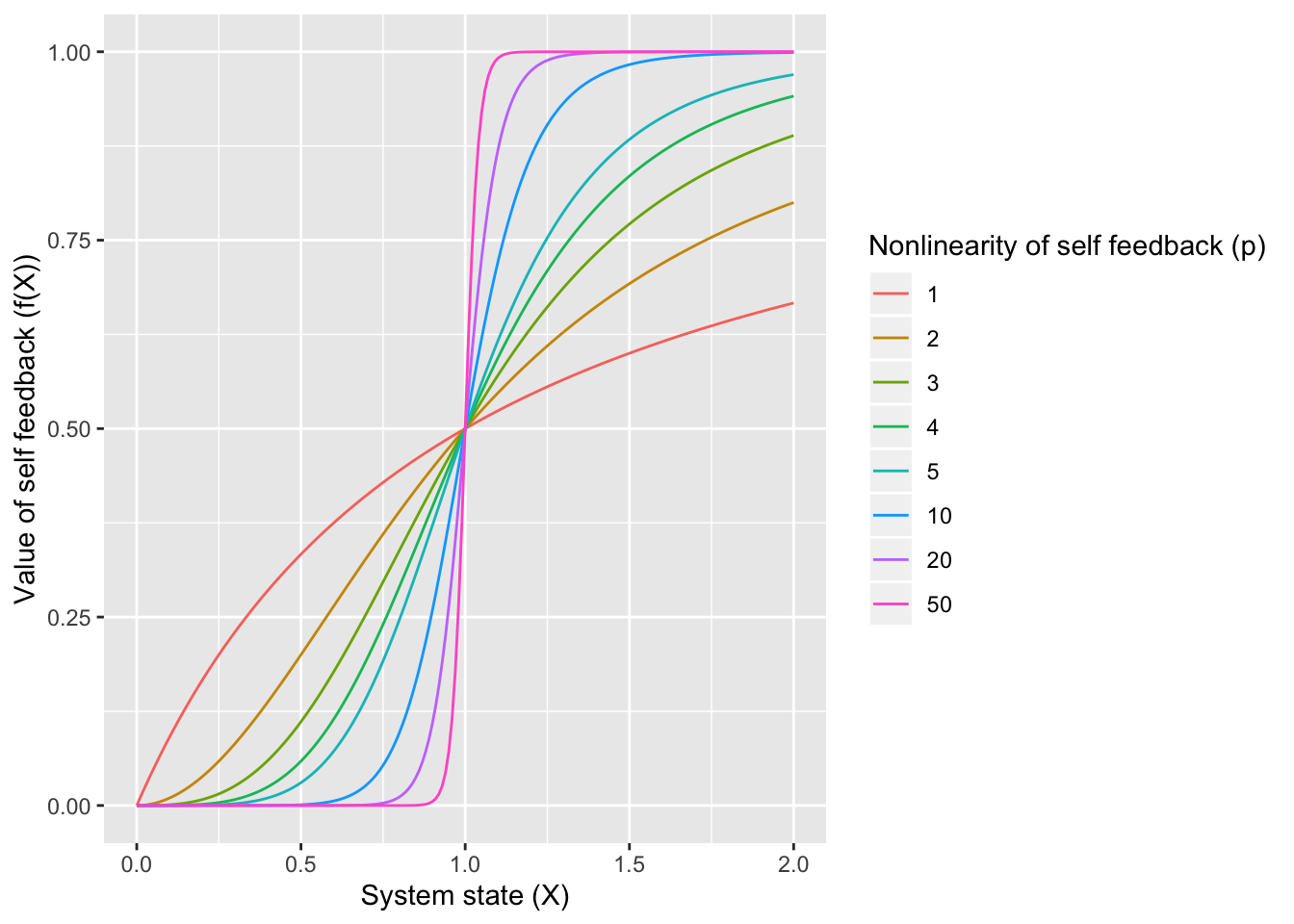# Introduction

The original paper by Scheffer et al (a review in Nature in 2001) describes how smooth changes in environmental conditions can create drastic / catastrophic changes in ecosystem state. These changes can display hysteresis, whereby responses to changing environmental conditions strongly depend on historical events. The paper reviews evidence of such shifts in lakes, coral reefs, woodlands, deserts, and oceans. It goes on to discuss emerging patterns and implications for management.

The initial purpose of this reproduction is to use the model in Box 1, described as a minimal model of an ecosystem showing hysteresis, to create versions of figures 1, 2, and 3. Some time series of dynamics might also be nice.

There is much more that could be added:

• Putting the model exploration into a shiny app.

• Some stochasticity in the model, to show how flips between states can be caused by chance.

• Examination of early warning signals.

# A minimal model of an ecosystem showing hysteresis

$$\frac{dx}{dt} = a - bx + rf(x)$$

$$x$$ is an “unwanted” ecosystem property. $$a$$ is an environmental factor that “promotes” $$x$$. $$b$$ is the rate of decay of $$x$$. $$r$$ is the rate at which $$x$$ recovers, as a function of $$x$$.

“For a lake, one can think of $$x$$ as nutrients suspended in phytoplankton causing turbidity, of $$a$$ as nutrient loading, and $$b$$ as nutrient removal rate, and of $$r$$ as internal nutrient recycling. For desertification, one could interpret $$x$$ as barren soil, $$a$$ as vegetation destruction, $$b$$ as recolonization of barren soil by plants and $$r$$ as erosion by wind and runoff.”

Hysteresis and alternative stable states can occur if $$f(x)$$ is a function with a threshold, e.g. the hill function:

$$f(x) = \frac{x^p}{x^p + h^p}$$

## Explore f(x), the function that determines the internal feedback

The non-linearity of this function is all important for the system dynamics. If the function is smooth (little non-linearity when $$p$$ is small) then the rate $$r * f(x)$$ varies smoothly with $$x$$ and dynamics are quite linear with the environmental condition $$a$$. If the function is very nonlinear (large $$p$$), then the system responds quite nonlinearly to changes in the environmental condition. Here is the function for some different values of $$p$$.# Figure 1: environment - equilibrium state relationships

Below are some relationships between environmental conditions ($$a$$) and equilibrium ecosystem state ($$x$$), going from smooth relationship (low values of $$p$$), to nonlinear relationship (medium values of $$p$$), to folded relationship (high values of $$p$$). Solid lines indicate a stable equilibrium, dashed lines an unstable one.

## Warning: cols is now required.
## Please use cols = c(roots)